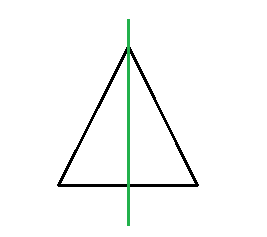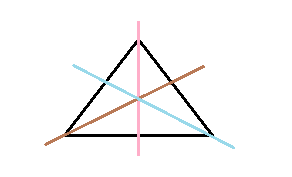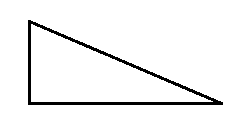# Can you draw a triangle which has (a) exactly one line of symmetry? (b) exactly two lines of symmetry? (c) exactly three lines of symmetry? (d) no lines of symmetry? Sketch a rough figure in each case.

(a) Yes.

An isosceles triangle can be drawn that has only 1 line of symmetry.(b) No, we cannot draw a triangle that has 2 lines of symmetry.

(c) Yes, An equilateral triangle can be drawn that has 3 lines of symmetry.(d) Yes

A scalene triangle can be drawn that has no line of symmetry.(1)(0)